# Module II, Week 1: Prelab #1

P1.1 Introduction
In this module we will be working with a rather cool piece of home-built equipment, an acoustic levitator! We will be doing far more than floating objects, however. We will also be honing one of the most important skills, some may say arts, of an experimentalist: performing accurate and precise measurements. This may not sound all that exciting at face value but it is an incredibly important part of experimental science. The GPS on my phone may be able to tell me my location on a map, but how useful would it  be if it was only certain to a radius of 5 miles? Or what if it was consistently reporting a location that was 1 mile to the east of my actual location? Understanding how well we truly know the value of something, being able to increase the accuracy to be useful for our application, and being certain that we are actually measuring what we think we are measuring are fundamental to good scientific practice.

P1.2 Learning objectives:
Upon completion of today’s prelab, you should be able to:

• identify and understand the differences between resolution and random uncertainties as well as systematic error
• use Colaboratory to create plots (with and without weighted fits) of your data
• understand the basic theory behind standing waves and acoustic levitators

P1.3 Uncertainty Basics¹
One of our goals as experimental scientists is to try to minimize the uncertainty when we perform a measurement. In lab we can of course increase our confidence in our measurement by collecting as much data as possible, although we must decide when we have reached a point of “diminishing returns.” We must be aware of how much variance there is in our data and how to quantify that variance. We want to be aware of what factors in our experiment are introducing the most significant uncertainty and determine if there is some way we can improve our experimental design within our laboratory constraints. During lab you will need to ask yourself what factors could influence the result of your measurement. Each of these factors can be thought of as working against our having perfect knowledge about a “measurand” (the quantity we want to measure) and adding to the overall uncertainty. A crucial aspect of experimentation is to identify the most important sources of uncertainty and to numerically estimate their effect on your measurement result.

Common sources of uncertainty include:

–the sensitivity of your instruments (e.g. the digital scale, smallest interval on a meter stick, etc.);
–the rating or stated calibration of the instrument;
–approximations and assumptions that you make while doing the experiment;
–the effects of environmental conditions on the measurement.

A measurement uncertainty is not meant to be an indication of “mistakes” that you might make in an experiment. If you are aware that you have made a mistake, then you should repeat your experiment or at least exclude the data taken improperly. Similarly, “human error” is not a useful way of identifying a source of uncertainty; that phrase provides future experimenters with no guidance at all about what aspects of the experimental apparatus or procedures to focus on improving.

P1.3.1 Sources of Uncertainty
There are many sources of uncertainty in your experiment, but not all originate in the same manner and therefore there are different methods of accounting for them.  When designing an experiment the aim is to make the uncertainty small enough to achieve the experiment’s goal — or often, to make the uncertainty as small as possible in order to observe as much detail as possible about the phenomenon.  However, always keep in mind that the uncertainty can never be reduced to zero, just made small and honestly estimated.

P1.3.1.a Resolution Uncertainty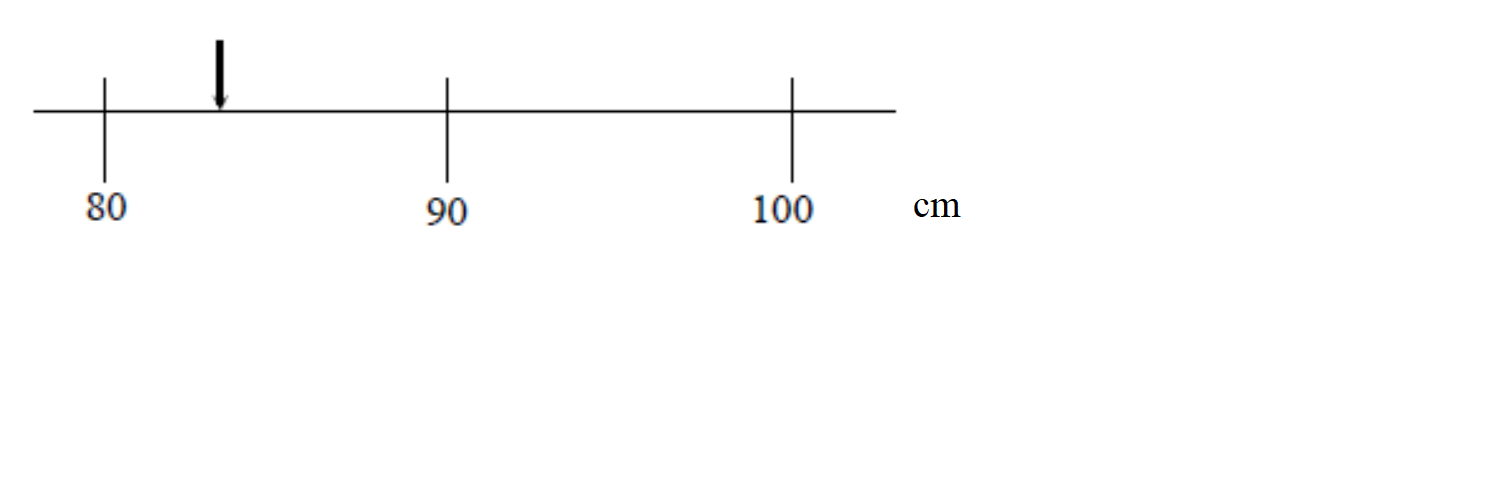Imagine you are attempting to measure the length of an object using the meter stick shown at the right. The length of the object is more than 80 cm but it is less than 90 cm but we are clearly limited by the resolution of our measuring device.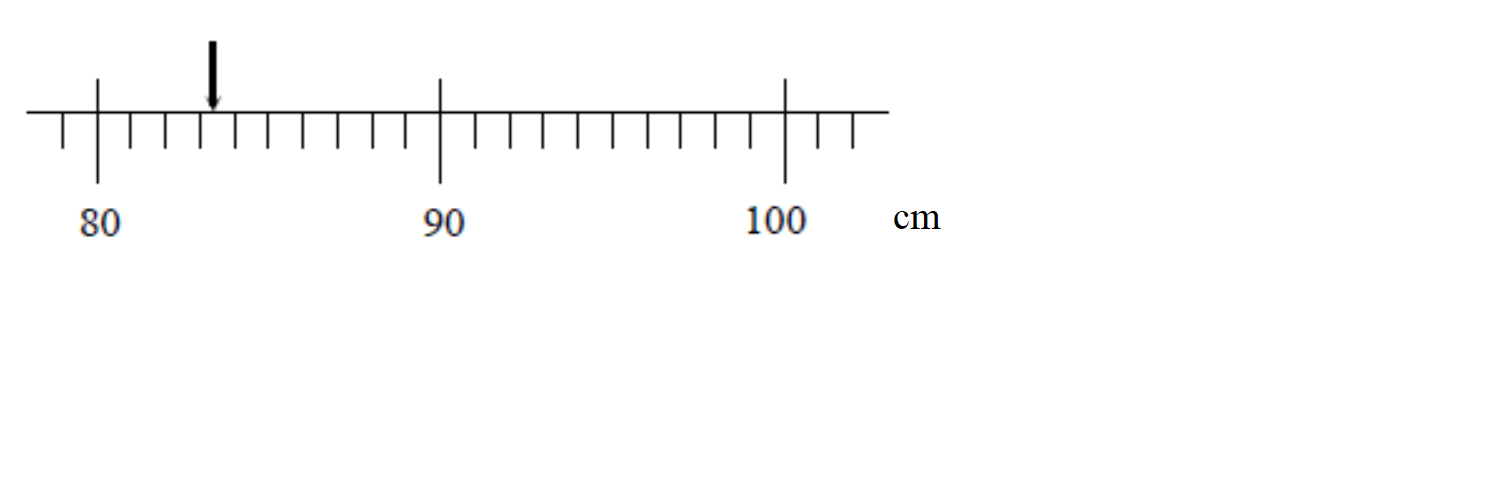We now get a better measuring device that has graduations down to the cm. We can now say that the length of the object is between 83 and 84 cm, although it appears to be closer to the 83 cm mark. Again, the accuracy of our measurement is limited by the markings on the meter stick.Finally, we obtain a measurement device that can measure much smaller intervals than our previous devices, surely we can now know the “exact” length of our object, right? As we see when we zoom in our measurement still could be improved by increasing the resolution of our measurement device!!

Apparently even with our super device there is a limit to the knowledge that the we can gain about the length of the object. All we can say is that the length of the object is between 83.4 and 83.5 cm. Even if we use smaller and smaller divisions on our meter stick our knowledge about the length of the object will never be perfect. This type of uncertainty is called the resolution uncertainty. A general rule of thumb is that the resolution is half of the smallest digit a device can measure, but it may be much greater than this if other known factors are limiting your uncertainty (perhaps you can’t get your ruler close enough to make an accurate measurement or can’t really tell where the object you are trying to measure begins or ends). Estimating uncertainties requires more than rules of thumb, it also requires judgement and common sense.

P1.3.1.b Random Uncertainty¹
In the last module you took measurements of the rotation rate of a motor. If you attempted to repeat your rotation rate measurement of a particular motor you most likely saw some spread in your values (you did not get an identical rotation rate each time you spun a motor under “identical” conditions). There will be slight and uncontrollable differences from one trial to another. These uncontrollable differences generally arise from a huge variety of detailed causes. Maybe the air conditioning happens to blow a slight puff of air on your setup the first time. Maybe a speck of lint falls on the sanded portion of the motor and reduces the current flowing through the motor. But, however these differences arise, they cause different results when a single procedure is repeated several times. The differences don’t trend in any particular direction, and their causes are subtle and hard to identify, let alone control, in the lab – so we call them random. These variations in data cause a spread in your data, as shown in the two histograms of repeated measurements from experiments A and B below: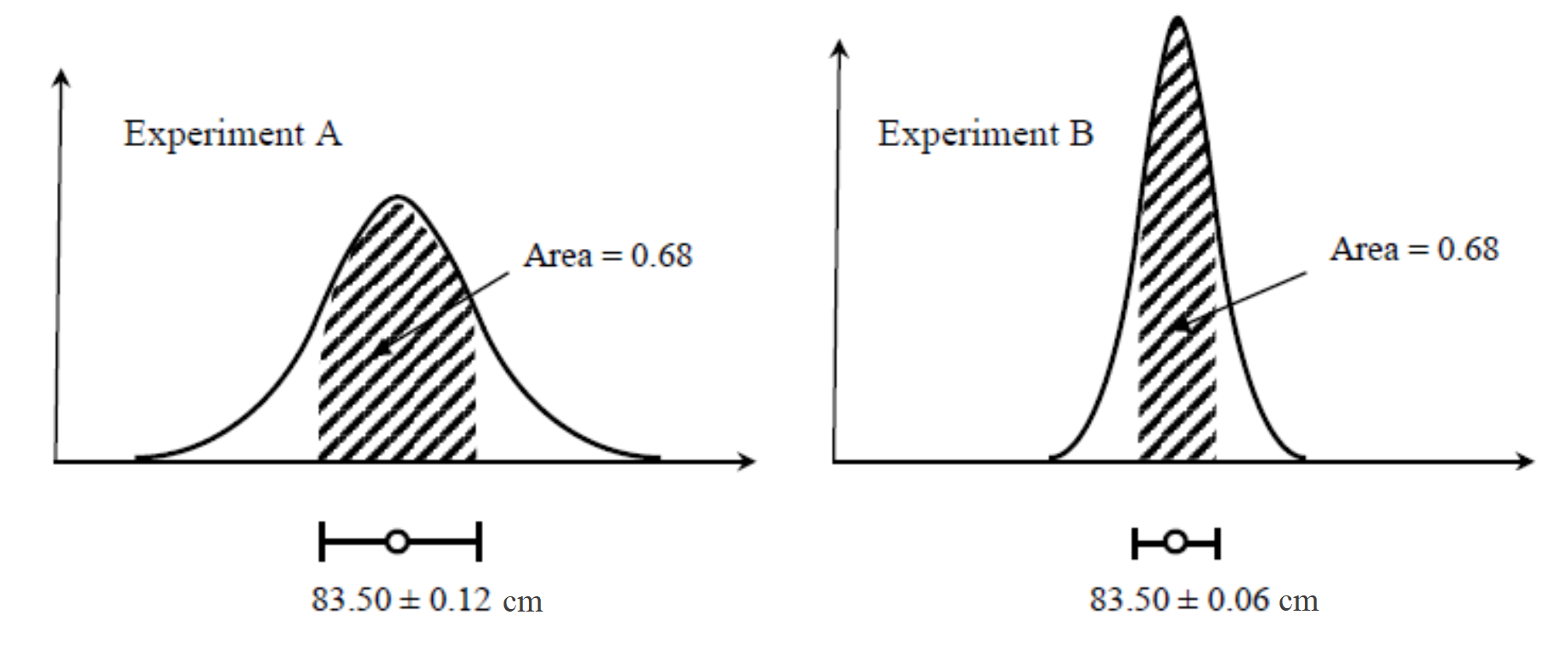Histogram of length measurements of a single object for two different experiments, A and B. What can you say about the difference in your confidence between the results of Experiment A and B?  (Area = 0.68 indicates in each case that the shaded area contains 68% of the total trials.)

Clearly, Experiment B has a narrower spread in the data and therefore has a lower uncertainty, but how do we calculate this uncertainty? It seems reasonable that the result of your multiple measurement trials would be the mean value $$\pm$$ some uncertainty that is related to the spread in your data. The range in which 68% of your measurements reside is the mean $$\pm \sigma$$ where $$\sigma$$ is the standard deviation of the data. However, taking more data will likely not decrease the value of the standard deviation of your data but it will surely increase your confidence in the mean and therefore should decrease the uncertainty. Therefore, the uncertainty we report will be $$\pm \frac{\sigma}{\sqrt{N}}$$, known as the standard error, where N is the number of trials (data points taken under “identical” experimental conditions).
Tip: Google Sheets can calculate the standard deviation of your data for you by using the function STDEV().  You can read more on this here.

Lesson check: If you take several trials of a particular measurement and record an identical value every time, does this mean you know the exact value with no uncertainty? What does this say about your experimental procedure?

P1.3.1.c Systematic errors
A group from another table in your section uses the exact same equipment and setup as you (i.e. same motor, oscilloscope, etc) to measure the rotation rate of the motor. They design their own, independent procedure to measure this quantity. The two groups can have differing results not only because of random uncertainty but also because of systematic error.

Systematic error arises when your experimental procedure and/or apparatus somehow cause all your measurements to be shifted away from the true value of the quantity you set out to measure. A systematic error happens in the same direction and the same (or similar) size in all your data, so its effect only shows up when an alternate measurement procedure is compared to yours.

For example, if you measure the length of an object with a meter stick that is 1.1 m rather than 1 m long (i.e. the spacing between mm marks is 10% too large) then all of your length measurements will be larger than those measured with a calibrated meter stick. Systematic errors are biases in the experimental process that need to be considered separately from resolution error and random uncertainty.  One good way to identify systematic error is to try several methods of collecting the same data.  If they should produce equal results (within resolution and random errors) but are consistently shifted from each other, then at least one method has a systematic error.  Another hint that you have a systematic error is to analyze your data for an expected shape or pattern.  If the basic pattern is what theory predicts but there is an offset, either the theory applies poorly to reality or you have a systematic error.  The best scenario is that you identify the source of systematic error and eliminate it from your process.  If that doesn’t happen, at least the size of systematic error can be estimated from careful checks like these.

Lesson check: what sources of systematic error could have been present in your experiment from the first module? How could you have tested this?

P1.3.2 Combining Uncertainties
When you make a measurement you will have both random and resolution (sometimes multiple!) uncertainties. In order to obtain one actual uncertainty to quote in your value you can combine the sources by adding in quadrature. Adding in quadrature means to square each value for your uncertainty, add them all together, and take the square root of the sum:

$$total \quad \Delta x = \sqrt{(\Delta x_1)^2+(\Delta x_2)^2+(\Delta x_3)^2_…}$$

Where $$\Delta x$$ is the total uncertainty in the measured value $$x$$ and $$\Delta x_1, \Delta x_2, \Delta x_3, …$$ are the various sources of uncertainty in the measurement of $$x$$ (e.g. random uncertainty, resolution uncertainty, etc).

Remember: Systematic errors should be dealt with separately, by eliminating all you possibly can and estimating the size of remaining shifts, in addition to pinpointing the specific effect they would have on the final analysis.  (Would they make the speed of sound come out too high?  Would they make a linear graph look quadratic?  etc.)

P1.4 Plotting in Colaboratory
Now that we have talked about how to take into account uncertainties, what do we do with them? It turns out that we can do a much better job of fitting a trendline to our data when we take the uncertainty of each data point into account. While Google Sheets is convenient for making quick plots of your data it is rather limited when it comes to fitting your data. In particular, it does not have the ability to weight your data by each point’s uncertainty when finding the best fit. We have written a script in Python, executed by a Google App “Colaboratory,” that will pull your data from your Google Sheet, plot, and fit it for you. It will also do simple plots of your data without a fit.

P1.4.1 Intro to Colaboratory
Follow the instructions below to plot your data with Python using the Google App “Colaboratory.”  Before you get started, watch the screencast demo below. Note: the Colaboratory file is now shared with you in Google Classroom rather than being accessed from this page. Colaboratory works best when using Google Chrome as your browser.

Colaboratory Tutorial

P1.4.2 Basic plotting of data
Using the files assigned to you in “Module II, Prelab 1” in Google Classroom, use the Colaboratory template to plot the sample data “Module 2: Voltage and Current sample data.” Complete the following steps to create a plot of Voltage vs Current.

– Open the Colaboratory template

– Open the section “Do this first” by either clicking on the black arrow or double clicking in the gray section just below the text.

– Run each of the three “Do this first” sections, from top to bottom, by clicking on the play button that appears when you hover over the [ ]. In the second code box you will be prompted to follow a link and connect your Google Drive account (see the above screencast). Click allow.

– Open the section “Now let’s plot your data,” open the section “Simple Plot (no fit or error bars)”

– This section defines the function “plot_no_fit” that you will use to plot your data below. When new data is added to your Google Sheet you will simply re-run the function rather than re-defining the parameters.
Fill in the following fields on the right hand side section with the white background (it will fill into the code section automatically):

spreadsheet: enter the exact name of your spreadsheet surrounded by ‘single quotes’ (note: do not give a path to the location in your Drive, just the exact name of the file)

worksheet_number: enter in the tab you would like to access on your spreadsheet, found in the lower left corner of your spreadsheet (the counting goes from left to right, beginning with zero)

x_values_column: column number on your spreadsheet that corresponds to the data you would like plot on the x axis (note: numbering goes from left to right, beginning with one).

y_values_column: column number on your spreadsheet that corresponds to the data you would like plot on the y axis

Plot_title: the title you would like for your plot

x_title: the title you would like for your x axis

y_title: the title you would like for your y axis

– Once all your values are entered run this code block (by clicking the play button that appears when you hover over the [ ]) in order to define the function “plot_no_fit.”

-Run the next code block in order to plot your data. Your data will be displayed above the plot so you can verify that you chose the correct columns for your x and y values.

– Once you are satisfied with your plot copy it by right-clicking (ctrl-clicking for Mac) and paste your plot into the Google Doc “Module II Pre-Lab-1” (shared with you in the pre-lab assignment in Google Classroom) in the section “Plot with no fit, no uncertainties.”

– Now we will plot your data and fit a trendline. Open the next section in Colaboratory “Plot and fit your data, no errors.” Follow the same steps outlined above to create a plot with a trendline.

– Once you are satisfied with your plot, copy paste it into the Google Doc “Module II Pre-Lab-1” in the section “Plot with fit, no uncertainties.”

P1.4.3 Plotting with Uncertainties
You may be wondering why we are bothering to teach you to use Colaboratory when, so far, you can do the same thing in Google Sheets. In this module we will begin to look at the uncertainties in our measurements and determine how these impact experimental conclusions.

In this case, we would like to get a value for the resistance of a circuit that takes into account the uncertainty in our measurements of V and I. In order to do this we will need to perform what is called a weighted fit. A weighted fit takes into account your confidence (uncertainty) in each individual data point. For example, a data point with 1% uncertainty will be given more importance than one with 10% uncertainty.

This capability is beyond the scope of Google Sheets and Microsoft Excel and we need to use a more sophisticated program. There are many options available but we have chosen Colaboratory since it uses Python, building upon knowledge you developed in your first year here at HMC.

– Open the spreadsheet that contains the sample data for this pre-lab. The second tab contains the same V and I data as before but we have now added a tab with uncertainties for your V measurement. (note: the fit weighting is only done using the uncertainties in the data plotted on the y-axis). We will discuss how to calculate errors this week in lab so just use our values for now.

– Under the section “Now let’s plot your data,” open the section “Plot your data with a weighted fit.”

– Enter the same parameters as you did in section P1.2.2.e. You will notice that there is now an additional field to enter the location of your uncertainty data. Use the number of the column that contains the uncertainties in V:

error_column: column number on your spreadsheet that corresponds to the uncertainties in the data plotted on the y axis.

-Run this cell (thereby defining the function “plot_with_fit”)

– Run the next cell in order to produce your plot with a fit. Notice that below your plot is the  following information about the line of best fit:

The linear fit equation is: $$y = b+ m * x$$
The uncertainty in the y-intercept is $$\Delta b$$
The uncertainty in the slope is $$\Delta m$$

where $$b, \Delta b, m$$ and $$\Delta m$$ are given numerically.

– Once you are satisfied with your plot copy and paste it into the Google Doc “Module II Pre-Lab-1” in the section “Plot with fit, with uncertainties.”

Now that we have an equation for a line of best fit for our data, what does it mean? Think about what these values mean physically in your system.

P1.5 Theory
Next week in lab you will be working with a standing wave of sound to levitate small objects (cool!). Let us take a few minutes to understand how they work.

P1.5.1 A Brief Review of Standing Waves
The acoustic levitators we will use in lab make use of standing waves of sound. Let us harken back to Ph 24 for a brief review of the basics of standing waves.

As you may recall, in a transverse wave the disturbance away from equilibrium, y, in a wave traveling in the x direction can be described by the equation $$y=Asin(k x-\omega t)=Asin(2\pi(\frac{x}{\lambda}-\nu t))$$ where $$A$$ is the maximum amplitude, $$\nu=\frac{\omega}{2\pi}$$ is the frequency, and $$\lambda$$ is the wavelength of the wave. The speed $$v$$ of the wave is given by $$v=\lambda \nu.$$

In the case of a wave on a string the wave travels along the length of the string and the disturbance y is the displacement of bits of string perpendicular to the length. Imagine an apparatus that consists of a string held under constant tension; one end is attached to a mechanical oscillator that jiggles the string, creating a sine wave that propagates towards the other end which is fixed (imagine it is tied to a post). The wave then hits the fixed end and reflects, creating a sine wave that propagates back toward the oscillator in the opposite direction. We now have two waves traveling in opposite directions and what we observe will be the result of these two waves added together.

Recall that the superposition of two waves traveling in opposite directions can be represented by $$y_{total}(x,t)=Asin(k x-\omega t) + Asin(k x+\omega t +\phi)$$ where the reflected wave has the same maximum amplitude as the incident wave but has picked up a phase $$\phi$$. Our combined wave can now be represented as $$y_{total}(x,t)=Asin(k x-\omega t) – Asin(k x+\omega t )$$ where we have made use of the fact that $$sin(\theta+\pi) = -sin(\theta)$$. Making use of one more fun fact, $$sin(A + B)=sin(A)cos(B)+sin(B)cos(A)$$, and a little bit of algebra you can show that this simplifies to $$y_{total}(x,t)=-2Asin(\omega t)cos(kx)$$

The takeaway from this is that the position and time dependence are now separate! It is as though the wave is fixed in space along the x axis while the effective “maximum amplitude” oscillates in time as $$2 A sin(\omega t)$$. This is known as a standing wave and is shown in the figure below.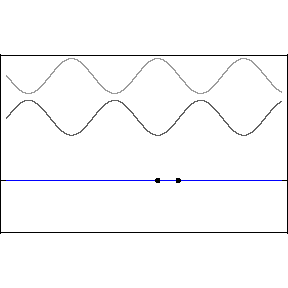Above is a  standing wave formed by counter-propagating sine waves. The blue standing wave is the sum of the upper two traveling waves. Animation courtesy of D. Russell

If both ends of the string are fixed (or nearly so), this puts restrictions on the wavelengths and therefore the frequencies that will produce stable standing waves on a given string. In this example, our string can be thought of has having two fixed ends –nodes —a distance L apart.  The places where the string doesn’t move ($$y_{total}=0$$) are called nodes, whereas places where the string oscillates with maximum amplitude are called antinodes. Only particular wavelengths will allow both ends of the string to be nodes; the wavelengths and frequencies that produce this type of stable standing waves are called resonant. (Note:  We have arrived at this condition by considering the boundary conditions for the disturbance at the ends of the string.  An equivalent method would be to require that the twice-reflected wave after one round trip is perfectly in phase with the brand new wave just now being emitted by the oscillator.  This second method offers some insight into what happens when the oscillator frequency is not resonant:  the vibrations on the string will be unstable and tend to die out.)

What happens if instead of a fixed end we have a second mechanical oscillator shaking the string at the same frequency? We will end up with a standing wave but the ends will not be nodes and it will not be defined by a resonant condition, meaning we are free to pick any frequency. This is the situation we have in the acoustic resonator and we will revisit it in more detail in the following section.

If you wish to revisit the above concepts, consult Appendix D of Motion II by Prof. Helliwell.

P1.5.2 Acoustic Levitator Theory
Air surrounds us all the time and it’s easy not to think about it at all, or to think of it as insubstantial.  But when a gust of wind blows over our beach umbrella, or a vacuum cleaner sucks up dust (or confetti, or popcorn…), it’s hard to ignore the fact that air molecules can exert force on other objects.  Specifically, these phenomena and many others involve net motion of many air molecules in response to a pressure difference.  In the case of the wind at the beach, the original pressure difference may be caused by weather patterns and by the contrasting temperature and motion of the water compared to the land.  In the case of the vacuum cleaner, the original pressure difference is caused by the vacuum action pulling air out of the canister.  Either way, air moves in response to the original disturbance, and can push solid objects as it goes.  Sound travels through air in a similar manner.  You bang on the table, and the vibrations in your hand and those on the table jiggle the nearby air.  This disturbance in the surrounding air creates a pressure wave in the air, which transmits outward and eventually vibrates your eardrums, which your ‘microphones’ (aka eardrums) interpret as a sharp bang.

Given that sound is motion of the air, might sound waves push and even levitate solid objects?  Indeed, this is the phenomenon of acoustic levitation, or ‘acoustophoresis,’ in which sound floats material objects.  The forces here are a bit more complicated than in the case of the beach umbrella or vacuum cleaner.  Both of those examples involved a steady or long-lasting pressure difference causing a sustained motion of the air in one direction.  As a sound wave passes through the air, however, the pressure in any given spot oscillates between high and low many times per second, but the vibrating air can still exert an overall force called acoustic radiation pressure.  We’ll discuss in more detail the various ingredients that go into acoustic levitation in a standing wave of sound.

First we’ll discuss the generation and propagation of a traveling wave of sound. Consider the following thought experiment. There is a speaker placed on a table, so that sound projects from the speaker to the ceiling. Suppose the speaker is attached to a function generator, and so outputs a noise with a constant frequency. The speaker operates by vibrating a plate’ up and down at the frequency set by the function generator, thus creating regular disturbances in the air, which your eardrums interpret as sound. These disturbances are longitudinal waves, which should be contrasted with transverse waves. Whereas a transverse wave vibrates in the direction perpendicular to the direction along which the wave is moving, longitudinal waves vibrate in the direction along the direction of motion of the wave. These distinctions are hopefully made clear by the following two GIFs. The left is a transverse wave while the right is a longitudinal wave like sound: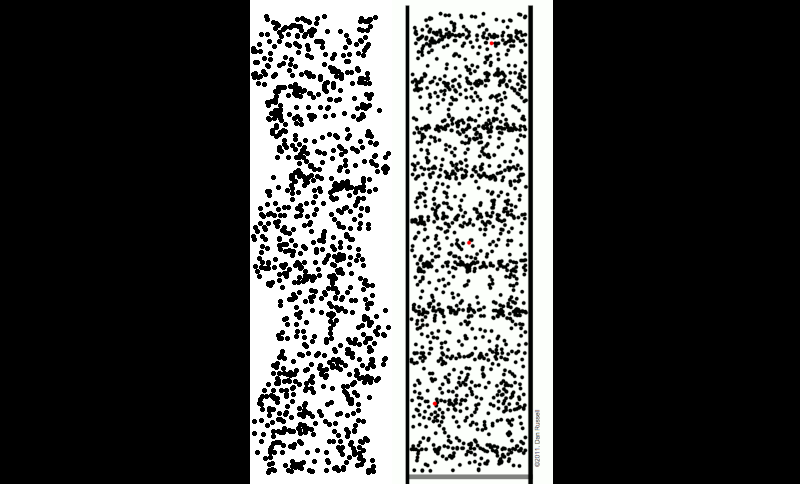Above are animations of transverse (left) and longitudinal (right) travelling waves. Note the difference in the behavior of individual particles in each wave type. Sound waves are longitudinal and look like the right-hand picture. Animation credit D. Russell.

Electromagnetic waves, as you will learn in Physics 51, are transverse. However, tsunami waves and slinky compression waves are longitudinal, as are sound waves.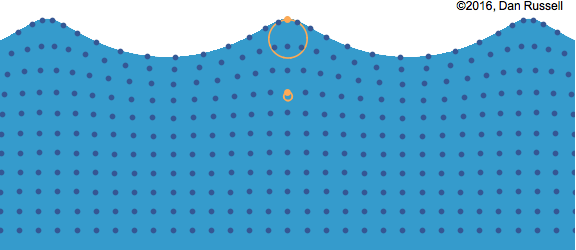Fun fact! The waves you see at the surface of the water in the ocean, those which break’ at the beach, are called surface or circular waves since particles in them effectively move in circles, and are neither longitudinal nor transverse, but rather a combination of the two as illustrated above.

Now, the longitudinal waves created above the speaker have a regular pressure pattern. When the plate in the speaker is forced downwards, the air directly above the speaker is ‘pulled’ apart, creating a low pressure, or “rarefaction,” region. But when the plate is accelerated upwards, the air above is ‘squeezed’ together, forming a high pressure, or “compression,” region. As individual air molecules move and collide, the pressure variations propagate upward as a travelling wave of sound.

We learned in the previous section that travelling waves of the same frequency, moving through the same material in opposite directions, can form a standing wave.  This can happen for sound as well.

The sinusoidal waves shown in the figure below represent the pattern of pressure variations in the air; they also looked exactly like the geometric shape of the string in our thought experiment above.  However, because sound waves are longitudinal, the actual motion of the air molecules looks geometrically a little different as shown in the figure below.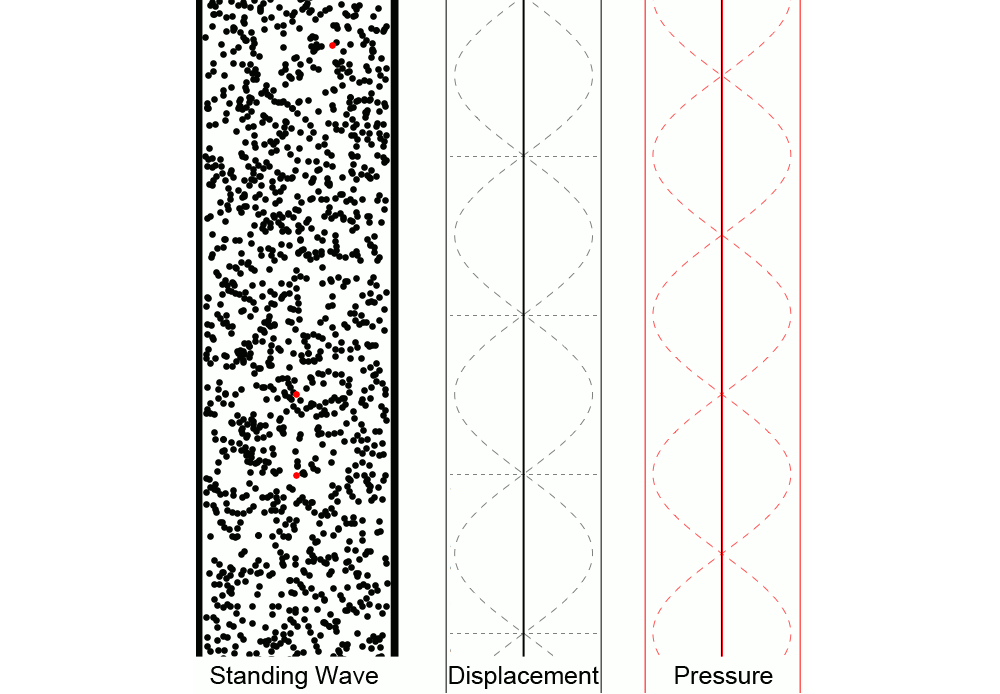The above animation shows a standing pressure wave that is oscillating at its resonance frequency. In the left animation we see the air particles being driven back and forth, creating regions of high and low pressure. The middle animation shows the displacement from equilibrium of a particle as a function of position. The right animation represents the deviation from equilibrium pressure as a function of position. Note this pressure wave is oscillating about the equilibrium pressure, not zero pressure. Notice the motion of the red particles for various locations and how the displacement and pressure animations relate to that motion.

In the previous section our standing wave on a string was caused by a traveling wave in one direction, driven by a source, and the reflections of this original traveling wave at the ends of the string.  A standing wave of sound can be created in the same way, using a single traveling-wave source like a speaker at one end of the setup and a sound reflector, like a metal plate, at the other.  Standing waves set up in this way are called resonant standing waves.  In a given setup, they are possible only at certain frequencies because the reflected waves have to interfere in just the right way with one another – or to put it another way, an integer number of half-wavelengths must fit along the length of the setup.  However, at the right wavelengths or frequencies, resonant standing waves can be very strong even if the original driving source is relatively weak; the reason is again that resonant standing waves involve constructive interference of the original wave with the waves that have gone before it and completed one round trip, or two round trips (etc.), through the setup.

Resonant standing waves of sound are very common.  (To play with some, consult a friend with just about any non-electronic musical instrument.)  However, our setup this week will involve non-resonant standing waves of sound.  Remember that the key ingredients for a standing wave are two traveling waves in opposite directions.  In a non-resonant standing wave, we simply place one source at either end of the setup.  If the two sources are driven together – by the same signal — they will produce traveling waves in opposite directions, of equal frequencies and amplitudes, creating a standing wave.  In a non-resonant standing wave, reflections of the original traveling waves are not crucial.  Therefore there are no specific frequency or wavelength requirements to make a standing wave ‘work’ in the setup (i.e., no harmonics).  However, since the original traveling waves don’t get ‘reused’ in the setup through multiple reflections, a non-resonant standing wave is generally not very strong unless the driving source itself is strong. You will see both of these features of non-resonant standing waves in the apparatus you will use this week.

In our acoustic levitator apparatus, the two sources are two sets of tiny speakers driven by the same electrical signal.  Each speaker set is arranged in a slightly concave bowl-like shape, and the two bowls face each other on opposite ends of a plastic frame.  The curved, concave shape of each source is a detail we haven’t yet discussed.  Its purpose is to shape the standing waves, making them focused and strongest in the middle of the apparatus.  The same thing is very commonly done for standing waves of light, with pairs of curved mirrors.  You can get a rough idea of how the curved surfaces produce curved wave patterns in the second half of this video.

How does a standing wave of sound produce acoustic levitation?  Take a good look at the standing wave in the previous figure .  A standing wave of sound consists of “pressure antinodes,” fixed positions in space where the pressure deviates the most from the equilibrium, atmospheric value, and “pressure nodes,” fixed positions in space where the pressure stays constant.  You might imagine a small styrofoam bead placed in the standing wave, being pushed away from a pressure antinode when the pressure is high there, and then being pulled toward it when the pressure is low there.  This doesn’t sound like it creates stable trapping!  However, the key to acoustic radiation pressure is that the standing waves oscillate too fast for a big, heavy particle (even a styrofoam bead) to follow the cycles of push and pull in a simple way.  Instead, the air in each region pushes the particle with an effective force proportional to the time average of the square of the pressure, $$<p^2>$$.  There is a well-developed theoretical foundation for this force   $$^2$$$$^3$$ but we will not go into it here.  What’s important to note for now is that $$<p^2>$$ is larger at the pressure antinodes than at the pressure nodes, so particles are consistently pushed either to the pressure nodes or to the pressure antinodes.  Most solid particles are pushed to (and trapped in) the pressure nodes; the exact details depend on the density of the object compared to air and the speed of sound through the object.

The exact details of this theory$$^4$$ are immaterial for us (it’s a good thing too, since it is very complicated!), the point is that we can discern the positions of the pressure nodes, and thus the locations at which (spherical) beads will levitate, using the above equation.

P1.6 Optional: DIY Acoustic Levitator!
If after this Module you are interested in building your own levitator you can find the AIP publication as well as a link to the materials and instructions below. Happy levitating!

___________________________________

$$^2$$ Gorkov, L. P., “Forces acting on a small particle in an acoustic field within an ideal fluid,” Dokl. Akad. Nauk SSSR 140(1), 88 (1961).

$$^3$$ A. Marzo, A. Barnes, and B. W. Drinkwater, “Tinylev: A multi-emitter single-axis acoustic levitator,” Rev. Sci. Instrum. 88(8), 085105 (2017).

$$^4$$ In the case of a perfectly rigid sphere with density $$>>$$density of air, the acoustic ‘levitation force’ between the two plates is encapsulated by something called the Gor’kov potential, given by $$U = 2\pi R^3\left(\frac{\langle p^2\rangle}{3\rho c^2} – \frac{\rho\langle u^2\rangle }{2}\right).\tag{eqn. 1}\label{eq:gorkov}$$ Here, $$R$$ is the radius of the levitating sphere, $$\rho$$ the air density, $$c$$ the speed of sound in the air, and $$\langle p^2\rangle$$ and $$\langle u^2\rangle$$ the mean square amplitudes of the sound pressure and velocity, respectively. Similar to Newton’s potential in orbital mechanics, or Coulomb’s potential in electrostatics, levitating objects subject to the Gor’kov potential reside in positions where (\ref{eq:gorkov}) is a minimum.